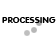Other products by CU Services
Item #: ES-27563
Our Price: \$349.00
Quantity:

## Description  more details

THE ENERGY ANALYST is a series of twenty-six sophisticated, engineering programs for the engineer who specifies, operates, or tests power plant components or evaluates energy alternatives. For more than 10 years, more than 2000 users have attested to the power and professionalism of these programs. And now with a complete conversion to the Windows format, each program has a friendlier, interface that will get you running in no time. The data handling techniques make your job easier, and although documentation is provided, it is seldom needed because the "fill-in" forms lead you by the hand at each step of the analysis The input data and the results can be printed with a single command and are in a form ready for inclusion in your final reports.
• Friendly user interface designed to get you running in no time
• Data handling techniques make your job easier
• Documentation provided
• Fill-in forms lead you by the hand through each step of the analysis
• Input data and results can be printed with a single command
• Input data and results and are in a form ready for inclusion in your final reports
• Calculates the maximum heating load required in a space (single zone) so that a furnace can be sized
• Calculates the performance of a known condenser, or can calculate the design requirements of a new condenser for a known steam load
• Analysis of induced, mechanical-draft, counterflow cooling towers, which can be used for the analysis of existing towers or for the preparation of specifications for new towers
• Calculates the performance of a steam, shell-and-tube heat exchanger of known construction using rigorous formulations
• Calculates the amount of steam that can be generated by a fired or unfired waste heat boiler
• Calculates the heat loss and surface temperature of an insulated pipe of specified diameter and internal temperature
• Analyze two types of heat exchangers: Shell & Multi-Tube Exchangers, and Double Pipe Exchangers
• Calculates the pressure loss of fluids flowing under pressure in circular pipes (compressible or incompressible)
• Uses the iterative Hardy-Cross algorithm to determine the flow and pressure loss in each leg of a series-parallel pipe network
• Uses the Manning equation to calculate the flow of water in sloped , circular drain lines
• Calculates the draft, friction loss, and exit loss for any boiler chimney
• Calculate either the flow for a give orifice size or, conversely, the orifice size for a given flow
• Design and analysis of duct systems transporting air, flue gas, etc.
• Calculates the amount of water needed for a known inlet steam flow or for a known outlet steam flow
• Calculates the performance of a steam turbine section given the inlet steam temperature, pressure, flow and the exit pressure
• Calculates the suction head, discharge head, power required, Net Positive Suction Head (NPSH) & suction specific speed for centrifugal pumps
• Computes the power required to compress any gas, the temperature required and the cooling required
• Analysis of expansion turbines
• Calculates the power required for a fan moving air or any kind of gas mixture
• Calculates the performance of a gas turbine using actual air enthalpy values
• calculates the amount of steam required to deaerate condensate at any pressure and temperature
• Calculates the the return on investment, present worth, and payback for a power plant that produces electrical power or produces both electrical power and heat energy
• Calculates the properties of superheated, saturated, or wet steam and saturated water
• Calculates the properties of a mixture of air and water vapor such as used in HVAC applications
• Calculates the economic results that can be achieved by using a flash tank and a heat exchanger to recover boiler blowdown
• Calculates boiler efficiency using the heat loss method of  the ASME Boiler Code PTC-4.1
• Fanno Flow Calculates the compressible, adiabatic flow of gases with friction.

Price: \$89.00
Price: \$89.00
Price: \$89.00

## Email A Friend